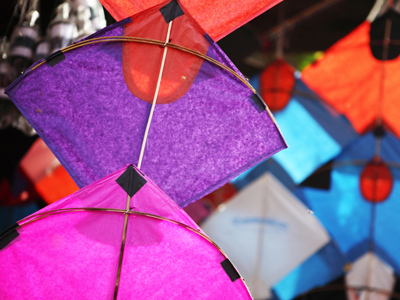A kite shape has two pairs of adjacent sides equal in length.

This Math quiz is called 'Shapes - Quadrilaterals' and it has been written by teachers to help you if you are studying the subject at middle school. Playing educational quizzes is a fabulous way to learn if you are in the 6th, 7th or 8th grade - aged 11 to 14.

It costs only \$12.50 per month to play this quiz and over 3,500 others that help you with your school work. You can subscribe on the page at Join Us

In the world of Math, quadrilaterals are two dimensional shapes that have four sides and four angles. There are different kinds of quadrilaterals, each with different properties.

1.
A scalene quadrilateral has no sides which are .......
curved
parallel
equal
smooth
2.
Here are four shapes. Which one is NOT a quadrilateral?
Kite
Parallelogram
Trapezium
Triangle
A triangle does not have four sides
3.
How many sides of a trapezium MUST be parallel?
2
3
4
None
4.
Which of the following does a parallelogram NOT have?
A line of symmetry
Four angles
Opposite angles are equal
Opposite sides which are equal in length
5.
What is a true statement about a kite?
All four sides are equal in length
Has one pair of parallel sides
Has two pairs of adjacent sides equal in length
It has no lines of symmetry
6.
Which of these shapes is NOT a quadrilateral?
Circle
Rectangle
Rhombus
Square
A circle does not have four sides
7.
What makes an isosceles trapezium different to an 'ordinary' trapezium?
A line of symmetry
No rotational symmetry
Non-parallel lines of equal length
One pair of parallel lines
8.
The interior angles of a quadrilateral add up to how many degrees?
90
180
360
720
9.
How many angles of a square MUST be 90 degrees?
2
3
4
None
All four sides MUST be equal too
10.
How does a rhombus differ from a parallelogram?
All sides are equal in length
Opposite angles are equal
Opposite sides are parallel
Rotational order of symmetry is two
Author:  Frank Evans